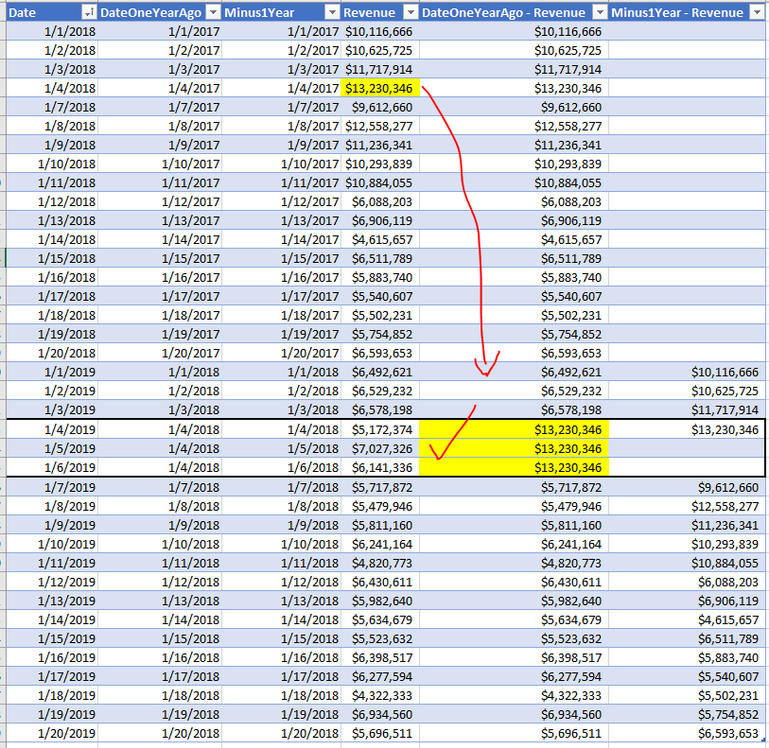cancel
Showing results for
Did you mean:Helper I

## Dax USERELATIONSHIP not working

I put together as a simple example of an issue that I'm having with the UseRelationship dax measure not working.

The problem is I have a date table where the date column is the unique key and we have a few more columns off to the side that map "Date" to its respective prior year date so that we can use the “UseRelationship” calculation to look at prior date values for a given date selected. In our dates table there are 2 additional columns that look back one year from the current date. One day called [Minus1Year] and is just subtracting one year from the date therefore there is a one to one relationship between date and minus one year. However, some dates are tagged to the same “date one year ago” value which is a separate column. For example, leap year. In the example I provide you will see that there are a few date rows that are mapped to the same “date one year ago” value and for the “minus one year” those values are unique and different. See highlighted example below. What is interesting is that if I build a dax measure that leverages the "date one year ago" relationship the relationship does not work because it is a many-Many relationship with the detail sales table, however, if there is a one to many relationship then the UseRelationship calculation works. As you can see in the example below I have a column for dates and I have a column for date one year ago which does have repeating values for some dates hence January 4th 2019 shares the same date one year ago with January 5th and January 6th of 2019.

The revenue column is simply is a sum of the revenue for the given date. No issue here….

Revenue = SUM(Sales[Amount])

• The "Date one year ago" is calculating the revenue amount but using the relationship between date one year ago from the dates table connecting to the transaction date column of the sales table. This calculation is not working properly and is only returning the revenue value. So you can see date one year ago revenue is equal to the revenue column.
• The minus one year revenue column seems to be calculating properly since that is a unique field from the dates table.

How do I fix the date one year ago revenue measure so that given a specific date it uses the respective date one year ago and returns the revenue value from that time similar to how minus one revenue works today. What I would expect to see is that on the values for January 4th 5th and 6th of 2019 they share the same revenue values since the “date one year ago” is the same for all three. Why isn’t this working and how can I get this to work?

Below are a few screenshots to help.

Here is the model. As you can see all fields from the dates table tie to the transaction date column of the sales fact table.Here is a view of the dates table. As you can see for some of the dates they share the same “date one year ago” value. This is done by design because some dates need to share the same “date one year ago” to account for leap year or other reporting dates.Here is a quick crosstab view showing by date and their respective “date one year ago” and “minus one year” columns. The values in the “DateOneYear – Revenue” are not correct and are simply equaling the Revenue column. As an example, below you can see for January 4th 5th and 6th of 2019, the "date one year ago" was January 4th 2018….so I would expect to see for each of these three rows in the date one year ago revenue column the value of \$13.2 million... unfortunately it's simply equaling the revenue. Why is this not working while the “Minus1Year – Revenue” column is working and how do I fix this?Here are the formulas for each of the 3 measures above. Hoping this is an easy answer….

DateOneYearAgo - Revenue = CALCULATE([Revenue],USERELATIONSHIP(Dates[DateOneYearAgo],Sales[TnxDate]))

Minus1Year - Revenue = CALCULATE([Revenue],USERELATIONSHIP(Dates[Minus1Year],Sales[TnxDate]))

Revenue = SUM(Sales[Amount])

Here are the expected results which I am not able to see...Thougths on what I am doing wrong and what is the fix for the "DateOneYearAgo - Revenue" measure?

1 ACCEPTED SOLUTIONSuper User

@powerbignc , for Time intelligence better to use date table and TI functions

example

Year behind Sales = CALCULATE(SUM(Sales[Sales Amount]),dateadd('Date'[Date],-1,Year))
Year behind Sales = CALCULATE(SUM(Sales[Sales Amount]),SAMEPERIODLASTYEAR('Date'[Date]))

Time Intelligence, DATESMTD, DATESQTD, DATESYTD, Week On Week, Week Till Date, Custom Period on Period,
Custom Period till date: https://youtu.be/aU2aKbnHuWs&t=145s

2 REPLIES 2Helper I

Year behind Sales = CALCULATE(SUM(Sales[Sales Amount]),dateadd('Date'[Date],-1,Year))

Here is the probem, I have another table that filters my Date table and when that table filters the Date table the "SAMEPERIODLASTYEAR" formula does not work... For some reason though it does work with the dateadd('Date'[Date],-1,Year).

So if you have a filter table filtering the Date table use dateadd NOT SAMEPERIODLASTYEARSuper User

@powerbignc , for Time intelligence better to use date table and TI functions

example

Year behind Sales = CALCULATE(SUM(Sales[Sales Amount]),dateadd('Date'[Date],-1,Year))
Year behind Sales = CALCULATE(SUM(Sales[Sales Amount]),SAMEPERIODLASTYEAR('Date'[Date]))

Time Intelligence, DATESMTD, DATESQTD, DATESYTD, Week On Week, Week Till Date, Custom Period on Period,
Custom Period till date: https://youtu.be/aU2aKbnHuWs&t=145sAnnouncements#### Exclusive opportunity for Women!

Join us for a free, hands-on Microsoft workshop led by women trainers for women where you will learn how to build a Dashboard in a Day!#### Power Platform Conference-Power BI and Fabric Sessions

Join us Oct 1 - 6 in Las Vegas for the Microsoft Power Platform Conference.Top Solution Authors
Top Kudoed Authors
Users online (3,279)